# Regular Grammars Generate Regular Languages 1 Theorem Regular

• Slides: 44Regular Grammars Generate Regular Languages 1Theorem Regular grammars generate exactly the class of regular languages: If is a regular grammar then is a regular language If is a regular language then there is a regular grammar with 2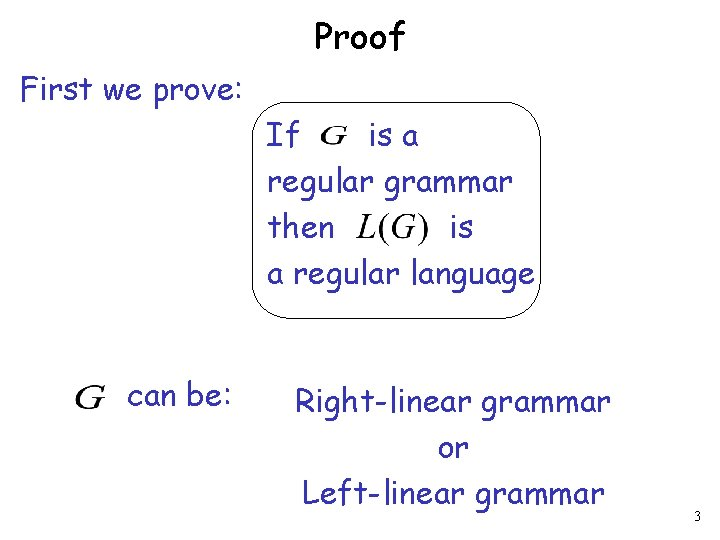Proof First we prove: If is a regular grammar then is a regular language can be: Right-linear grammar or Left-linear grammar 3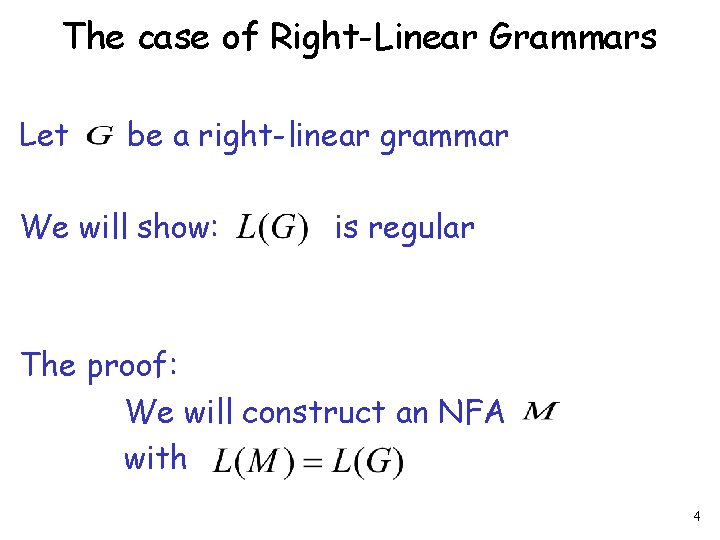The case of Right-Linear Grammars Let be a right-linear grammar We will show: is regular The proof: We will construct an NFA with 4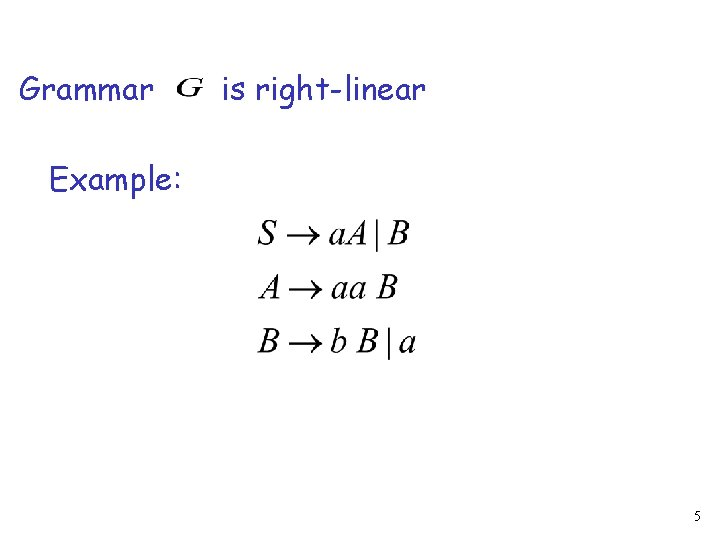Grammar is right-linear Example: 5Construct NFA such that every state is a variable: special final state 6Add edges for each production: 78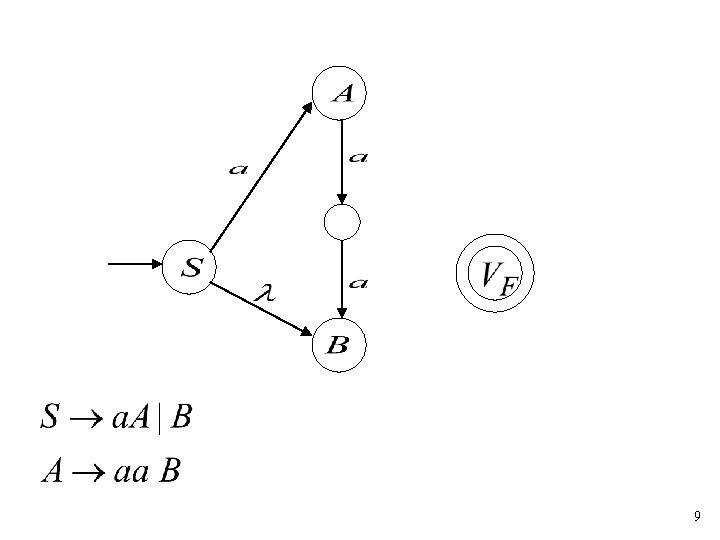91011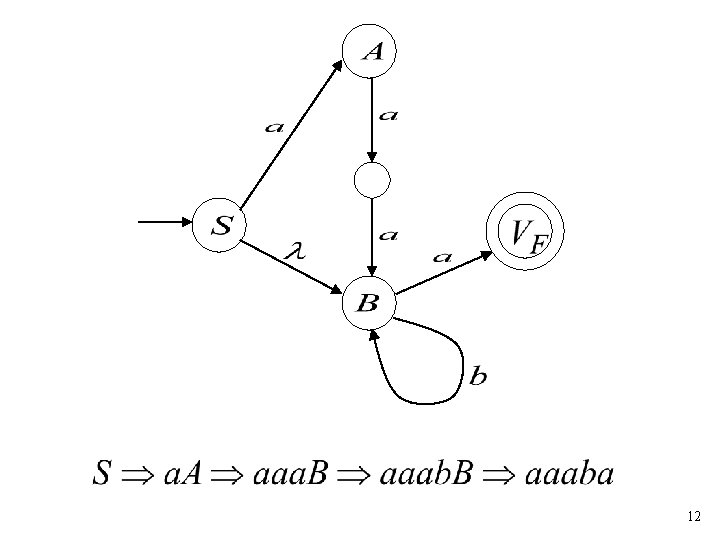12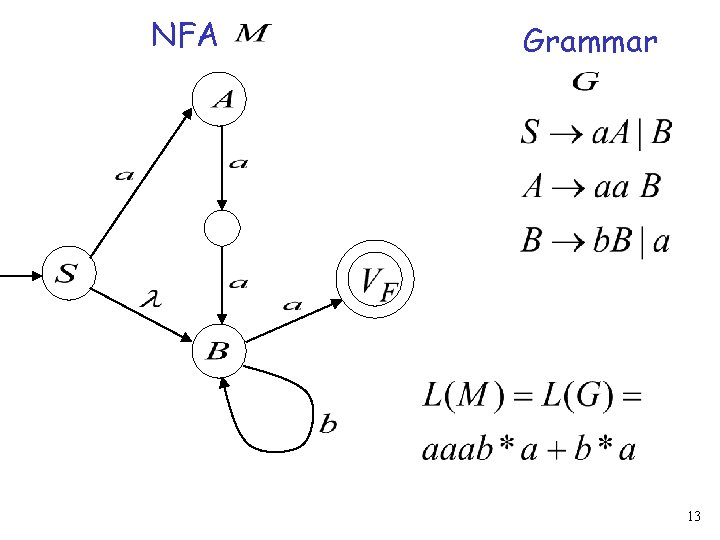NFA Grammar 13In General A right-linear grammar has variables: and productions: or 14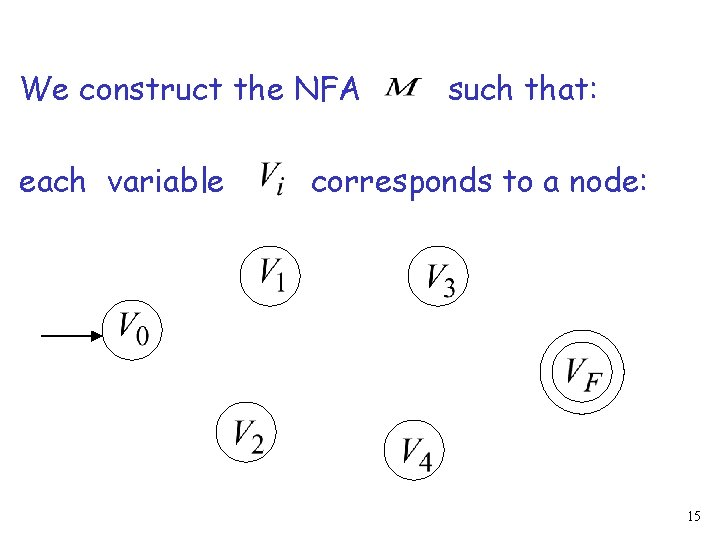We construct the NFA each variable such that: corresponds to a node: 15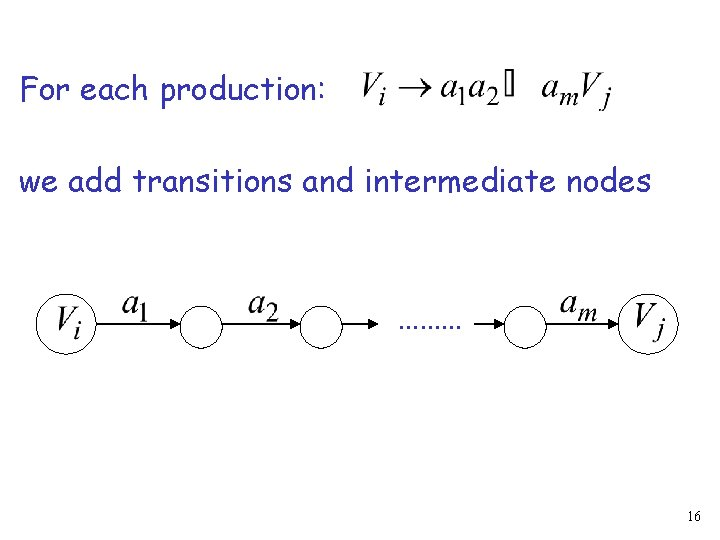For each production: we add transitions and intermediate nodes ……… 16For each production: we add transitions and intermediate nodes ……… 17Resulting NFA looks like this: 18Now, we need to show: 19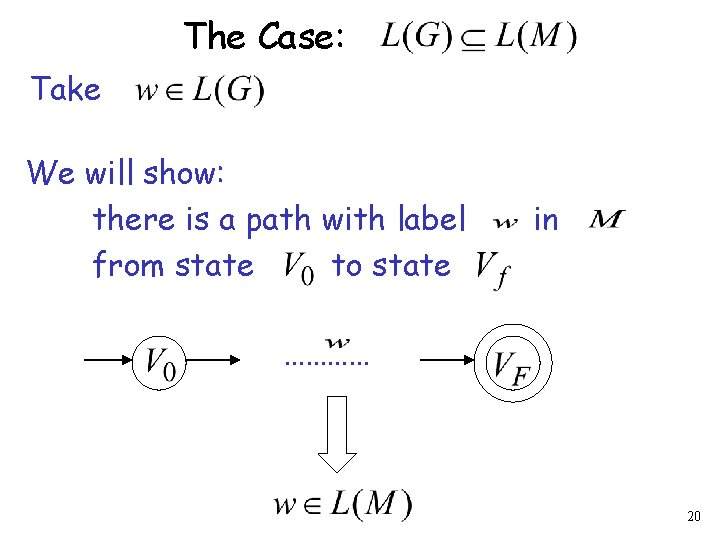The Case: Take We will show: there is a path with label from state to state in ………… 20Grammar looks like: strings 2122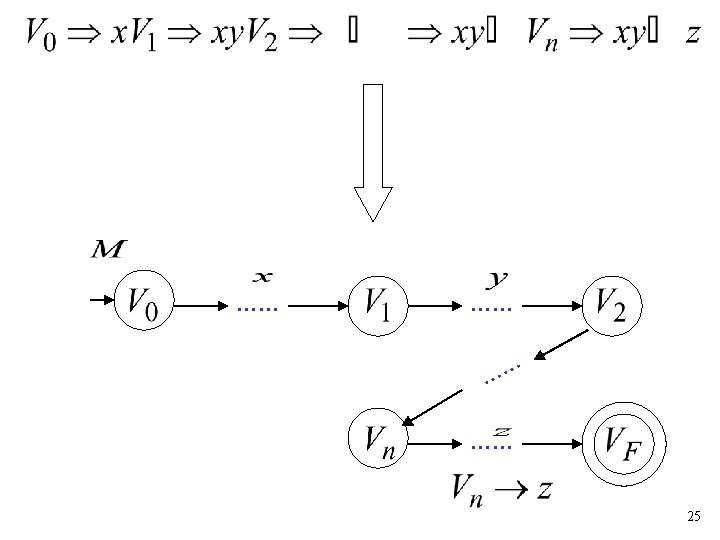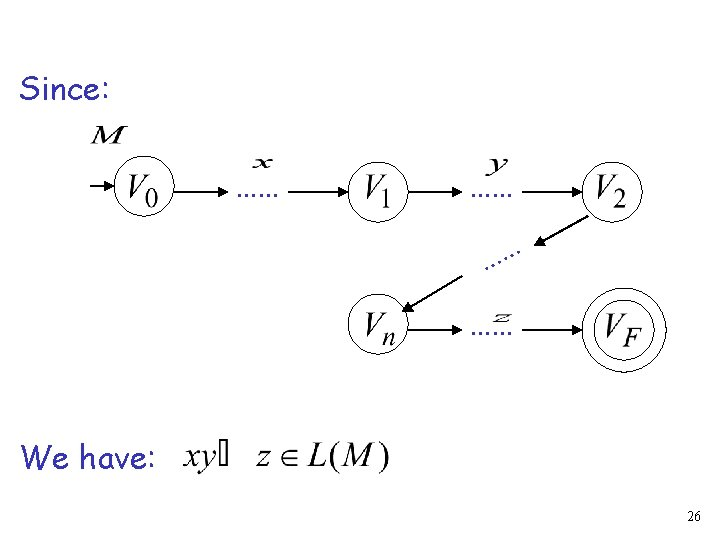Since: …… …… We have: 26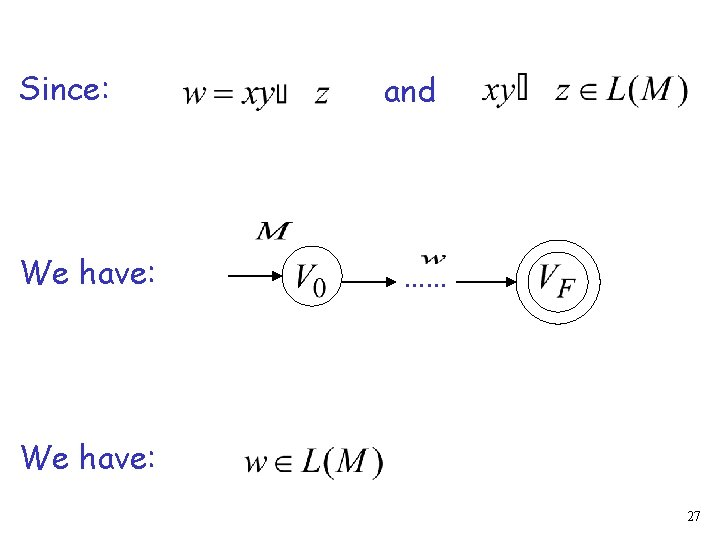Since: We have: and …… We have: 27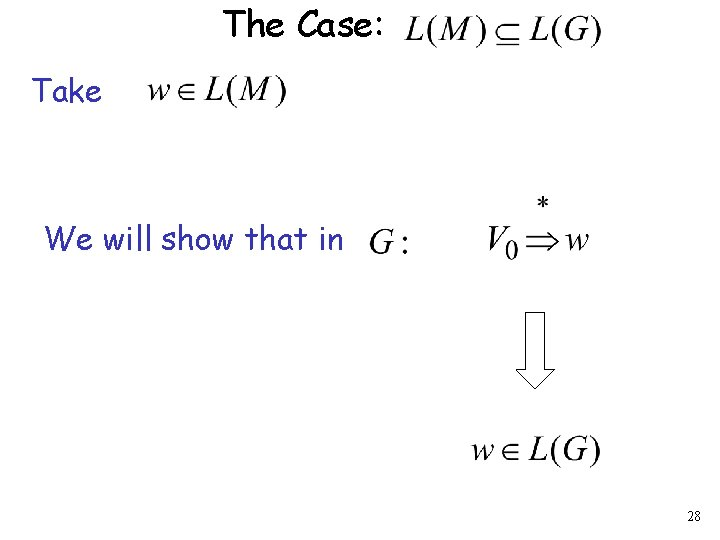The Case: Take We will show that in 28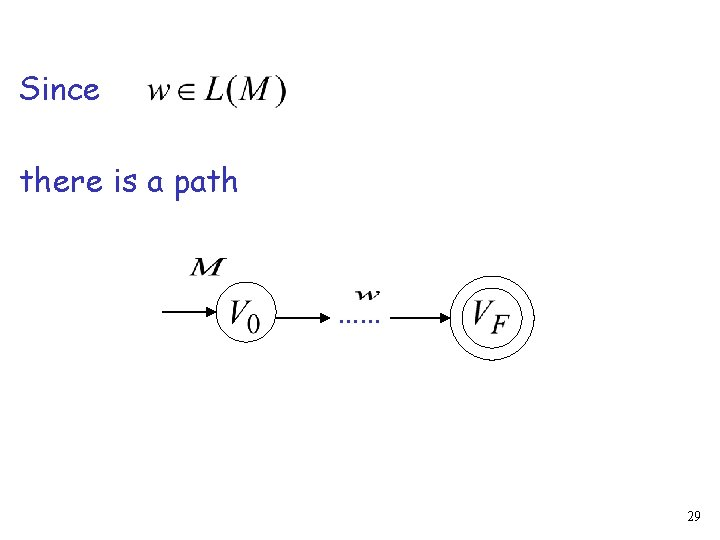Since there is a path …… 29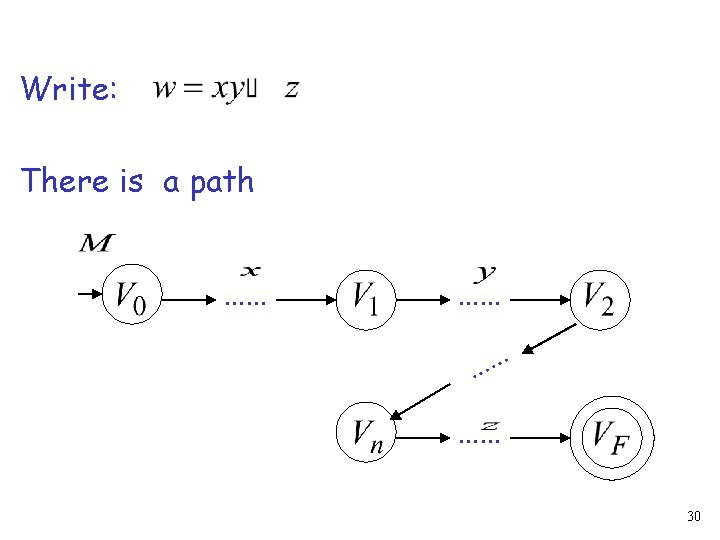Write: There is a path …… …… 30Since: …… …… This derivation is possible 31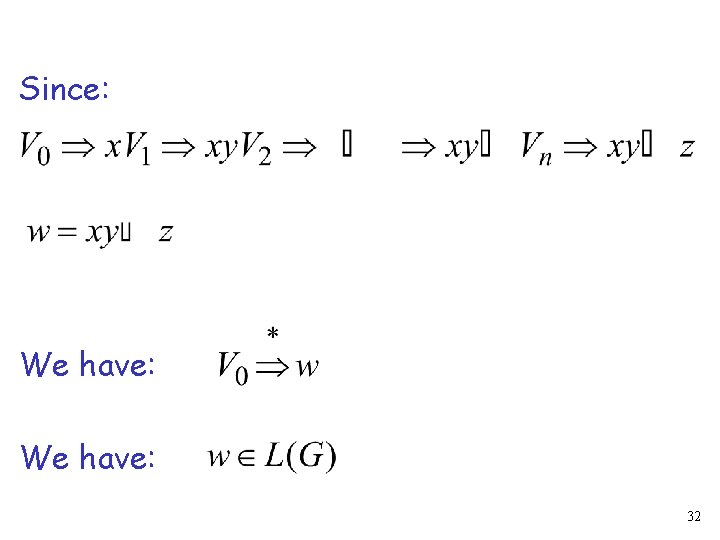Since: We have: 32The Case of Left-Linear Grammars Let be a left-linear grammar We will show: is regular The proof: We will construct a right-linear grammar with 33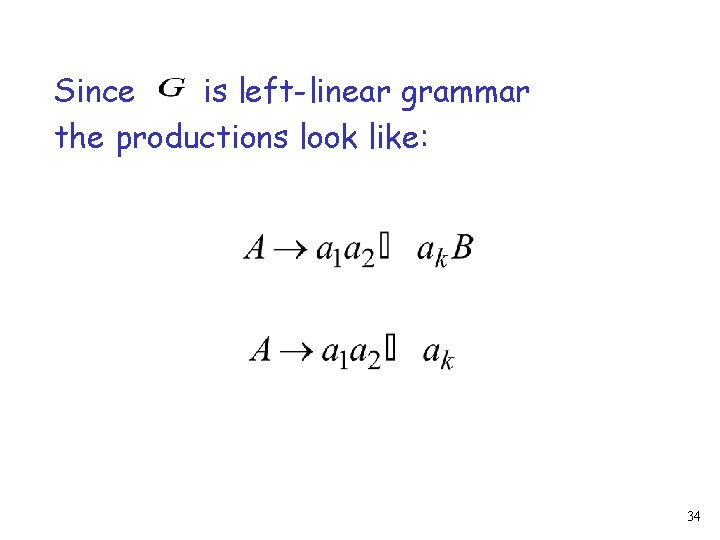Since is left-linear grammar the productions look like: 34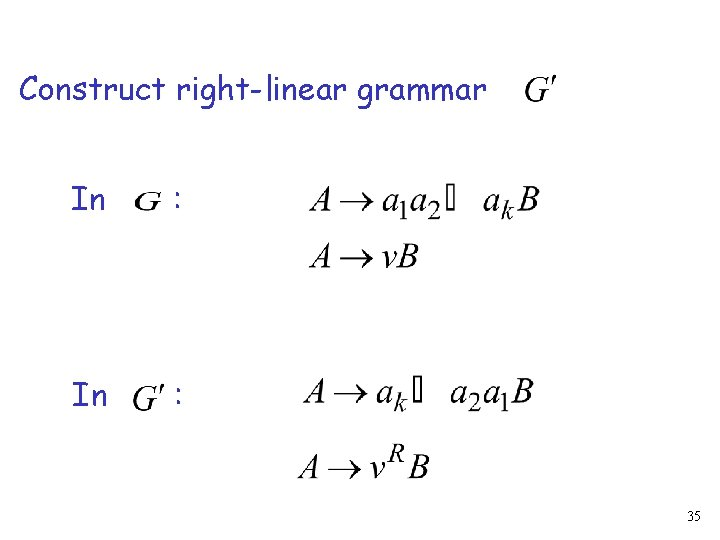Construct right-linear grammar In : 35In : 36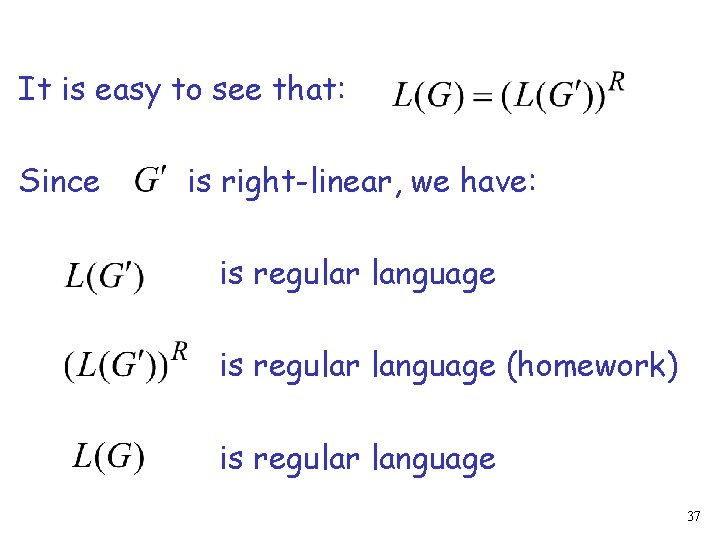It is easy to see that: Since is right-linear, we have: is regular language (homework) is regular language 37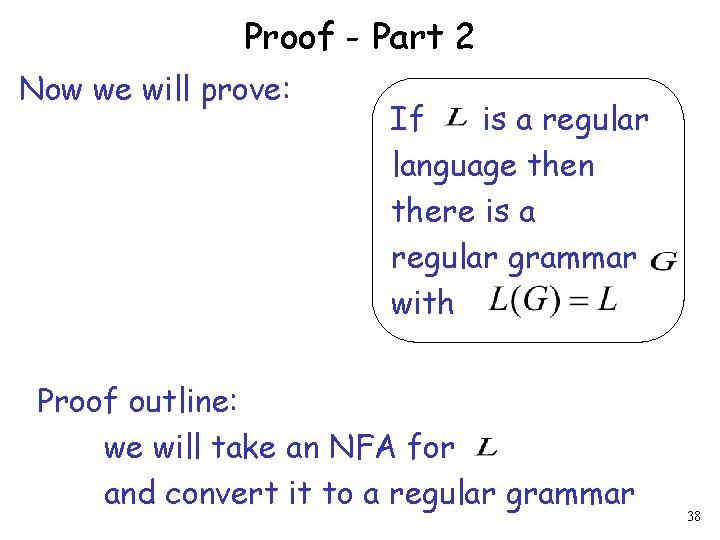Proof - Part 2 Now we will prove: If is a regular language then there is a regular grammar with Proof outline: we will take an NFA for and convert it to a regular grammar 38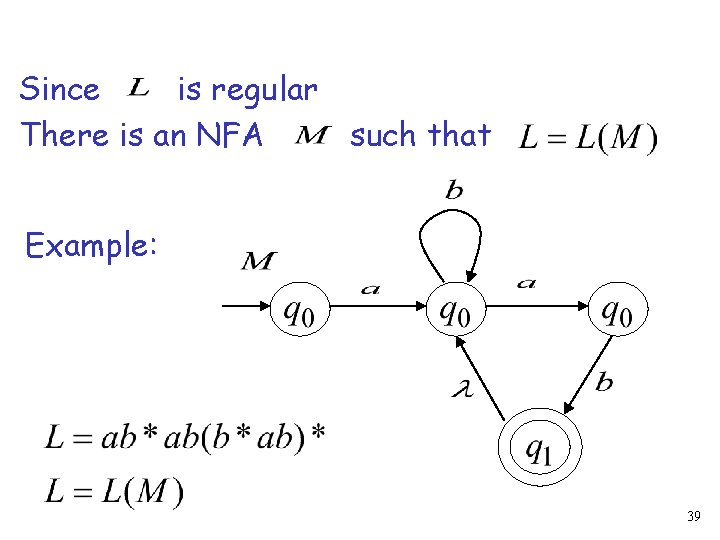Since is regular There is an NFA such that Example: 39Convert to a right-linear grammar 404142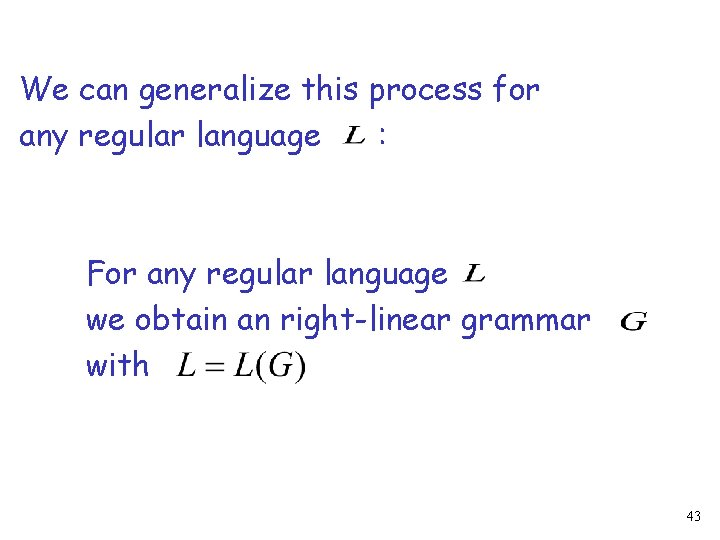We can generalize this process for any regular language : For any regular language we obtain an right-linear grammar with 43Since is right-linear grammar is also a regular grammar with 44